# A review of Dropout as applied to RNNs part 2.

Results and analysis.

In part 1 of this post I gave an overview of a brief history of dropout as applied to recurrent neural networks (RNNs). In part 2 I will show results of analysis of the importance of various dropout types on RNNs.

The dropout parameters tested here are the five dropout types applied in AWD-LSTM for language modelling tasks and four dropouts applied to different parts of a sequence to sequence attention model used in translation tasks.

Language Modelling

Language modelling was conducted on the following datasets: Penn Tree Bank and Wikitext-2, while the translation task was conducted for French to English translation. The source code for the background to the translation modelling can be found here.

In the two figures below I show the results on the sensitivity of resultant validation loss to variation of each dropout parameter where other dropout parameters are set to default values. The results plotted are final loss after 100 epochs.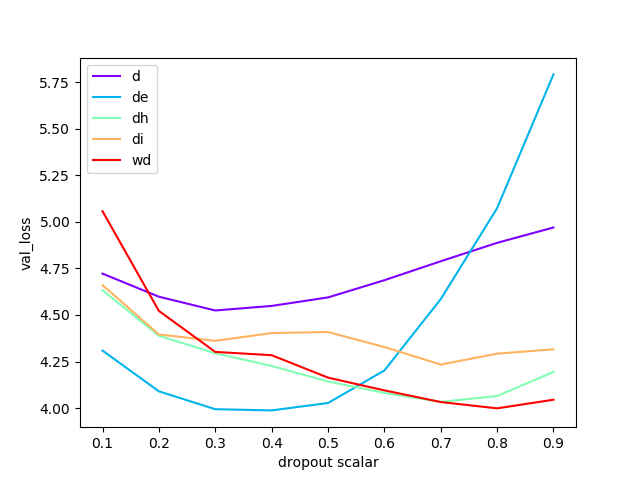Fig. 1. Fastai implementation of AWD-LSTM. Sensivity of validation loss to variation of each dropout parameter when the other dropout parameters are kept at default values. Final loss after 100 epochs.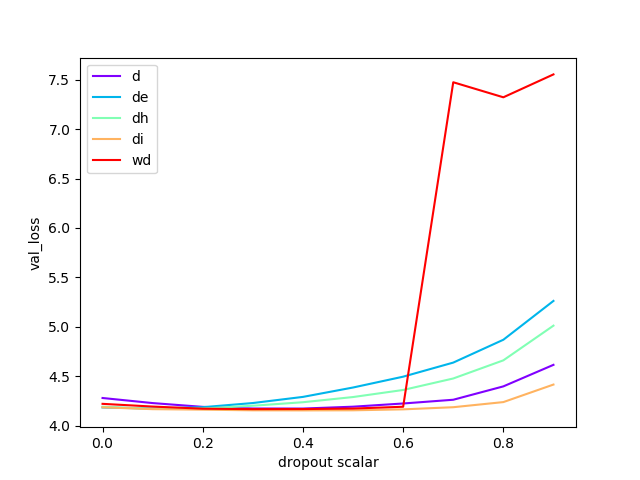Fig. 2. awd-lstm-lm original implementation of AWD-LSTM. Sensivity of validation loss to variation of each dropout parameter when the other dropout parameters are kept at default values. Final loss after 100 epochs.

It is evident that in the awd-lstm-lm original implementation of AWD-LSTM weight drop > 0.6 causes a dramatic increase in validation loss.

In the results below I show tests where each dropout parameter in-turn was varied between 0 and 0.9, with all other dropout parameters set to zero.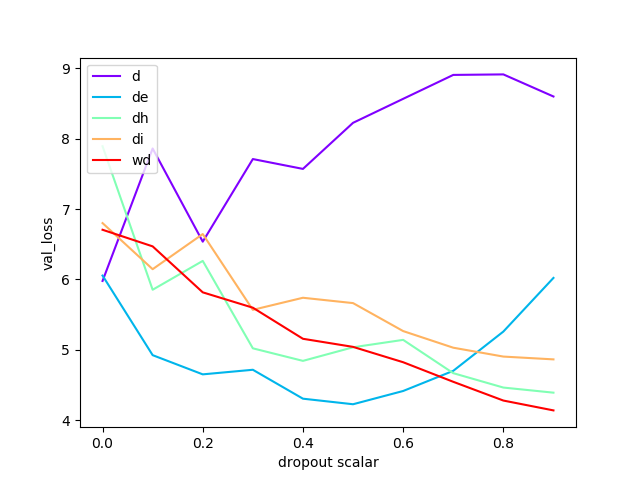Fig. 3. Fastai implementation of AWD-LSTM. Sensivity of validation loss to variation of each dropout parameter when the other dropout parameters are kept at zero. Final loss after 100 epochs.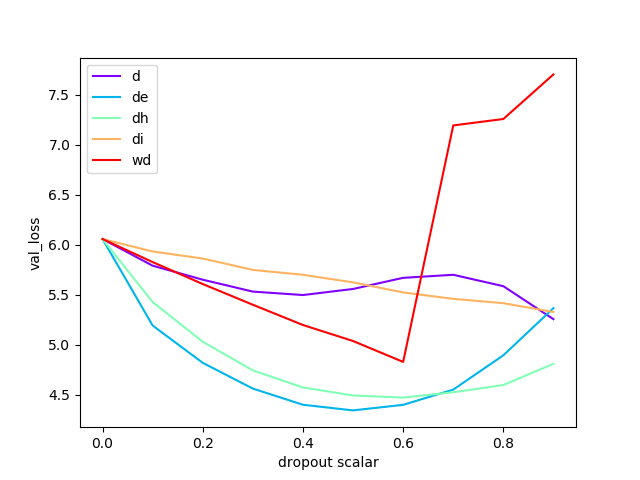Fig. 4. awd-lstm-lm original implementation of AWD-LSTM. Sensivity of validation loss to variation of each dropout parameter when the other dropout parameters are kept at zero. Final loss after 100 epochs.

Again we see a marked loss of performace for the awd-lstm-lm original implementation of AWD-LSTM where weight drop is > 0.6.

I repeated the analyses but just running for 12 epochs, as this is around the number of epochs often used in preliminary analysis. Note that the results below show dropout starting at 0.1 (not 0 as above) for all parameters.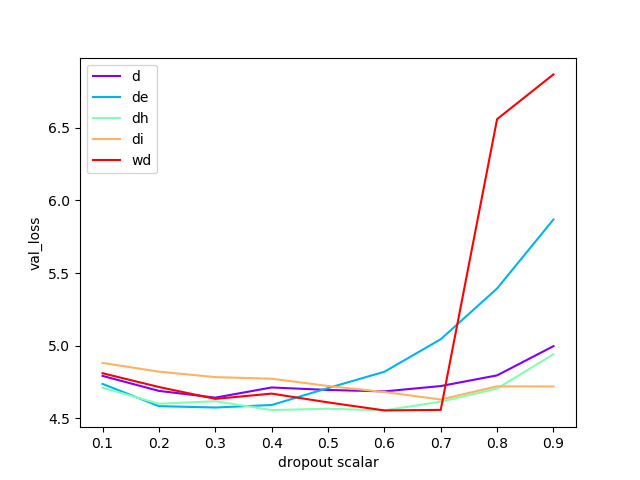Fig 5. awd-lstm-lm original implementation of AWD-LSTM on PTB dataset. Sensivity of validation loss to variation of each dropout parameter when the other dropout parameters are kept at zero.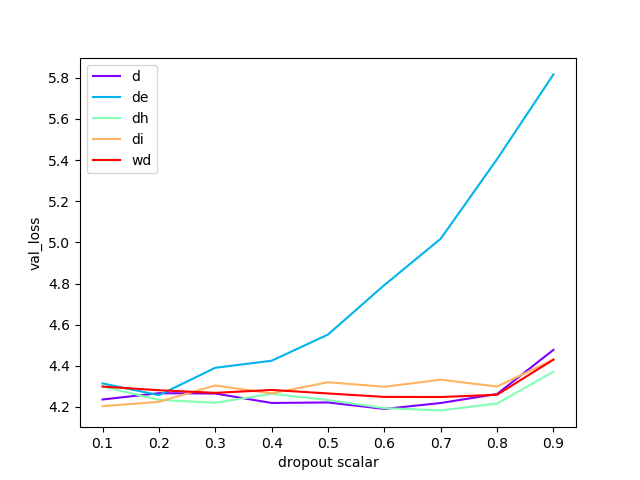Fig 6. Fastai implementation of AWD-LSTM on PTB dataset. Same analysis as per above. Note Weight Drop here was set to 0.001 for all tests except wd testing.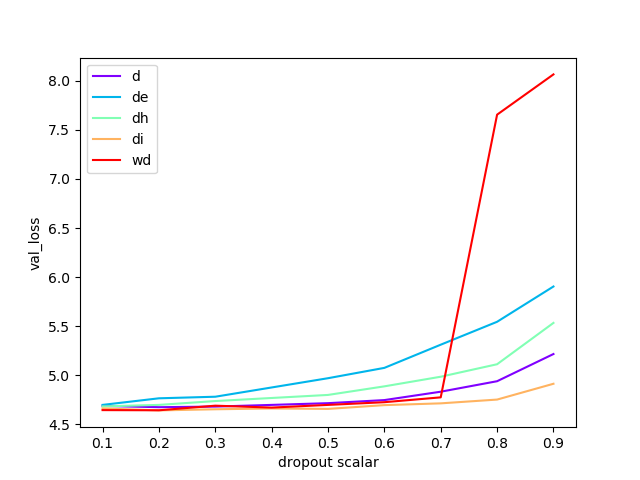Fig 7. awd-lstm-lm original implementation of AWD-LSTM on Wikitext-2 datset. Sensitivity of validation loss to variation of each dropout parameter when the other dropout parameters are kept at zero.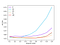Fig 8. Fastai implementation of AWD-LSTM on Wikitext-2 dataset. Same analysis as per above. Note Weight Drop here was set to 0.001 for all tests except wd testing (The fastai code has since been modified to allow a zero wd to be used).

Differences in code implementation on the AWD-LSTM vs fastai library for Weight Drop manifest in a clear difference in results at wd≥ 0.7, however all other parameter variation exhibit similar loss sensitivity.

Each dropout parameter is described below.

Embedding dropout (dropoute, abbreviated here as de) applies dropout to remove words from the embedding layer. “Following Gal & Ghahramani (2016), we employ embedding dropout. This is equivalent to performing dropout on the embedding matrix at a word level, where the dropout is broadcast across all the word vector’s embedding. The remaining non-dropped-out word embeddings are scaled by 1−pe where pe is the probability of embedding dropout. As the dropout occurs on the embedding matrix that is used for a full forward and backward pass, this means that all occurrences of a specific word will disappear within that pass, equivalent to performing variational dropout on the connection between the one-hot embedding and the embedding lookup.” Merity et al. (2017)

The code for embedded dropout from the awd-lstm-lm codebase can be seen below.

`def embedded_dropout(embed, words, dropout=0.1, scale=None):  if dropout:    mask = embed.weight.data.new().resize_((embed.weight.size(0), 1)).bernoulli_(1 - dropout).expand_as(embed.weight) / (1 - dropout)    mask = Variable(mask)    masked_embed_weight = mask * embed.weight  else:    masked_embed_weight = embed.weight  if scale:    masked_embed_weight = scale.expand_as(masked_embed_weight) * masked_embed_weight  padding_idx = embed.padding_idx  if padding_idx is None:      padding_idx = -1  X = embed._backend.Embedding.apply(words, masked_embed_weight,    padding_idx, embed.max_norm, embed.norm_type,    embed.scale_grad_by_freq, embed.sparse  )  return X`

This dropout has the most significant impact on network performance (excepting tail end WeightDrop on AWD-LSTM).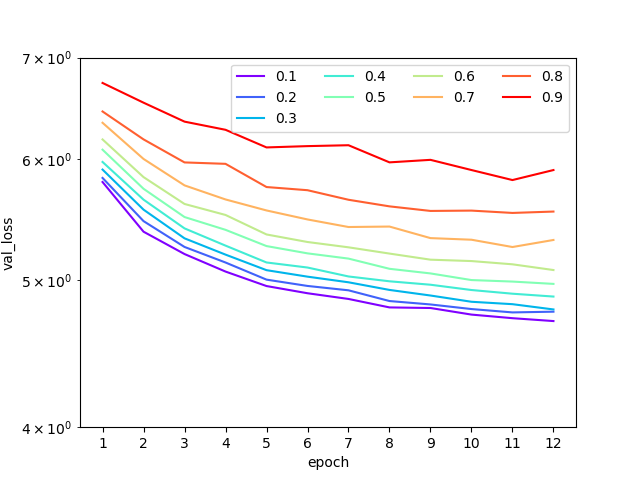Fig 9. awd-lstm-lm original implementation of AWD-LSTM varying Embedding Dropout (de) parameter on a) PTB dataset and b) Wikitext-2 datset. Other dropout parameters are kept at default values.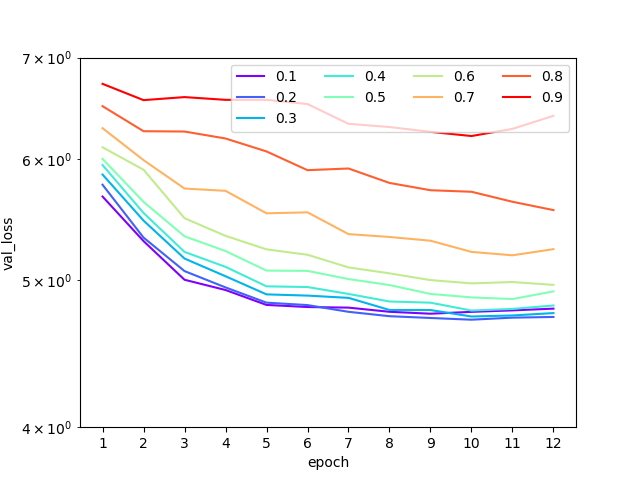Fig 10. awd-lstm-lm original implementation of AWD-LSTM varying Embedding Dropout (de) parameter on a) PTB dataset and b) Wikitext-2 datset. Other dropout parameters are kept at zero.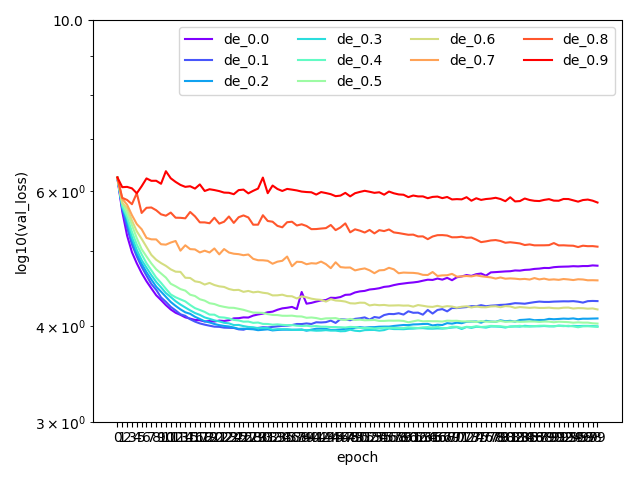Fig 11. a) AWD-LSTM (original) varying Embedding Dropout (de) parameter on PTB dataset. Other dropouts kept at default values. b) Fastai implementation of AWD-LSTM varying Embedding Dropout (de) parameter on PTB dataset. Other dropouts kept at default values.

Input dropout (dropouti abbrv. di) is applied in AWD-LSTM straight after embedding dropout in RNNModel(). Hidden dropout (dropouth abbrv. dh) is applied for each RNN, for each layer in the RNN and Dropout (dropout abbrv. d) is applied to the RNN output, after looping each RNN and each layer in them, dropout to the output as can be seen in the forward method of RNNModel in the awd-lstm-lm codebase.

`def forward(self, input, hidden, return_h=False):    emb = embedded_dropout(self.encoder, input, dropout=self.dropoute if self.training else 0)    emb = self.lockdrop(emb, self.dropouti)    raw_output = emb    new_hidden = []    raw_outputs = []    outputs = []    for l, rnn in enumerate(self.rnns):        current_input = raw_output        raw_output, new_h = rnn(raw_output, hidden[l])        new_hidden.append(new_h)        raw_outputs.append(raw_output)        if l != self.nlayers - 1:            raw_output = self.lockdrop(raw_output, self.dropouth)            outputs.append(raw_output)    hidden = new_hidden    output = self.lockdrop(raw_output, self.dropout)    outputs.append(output)    result = output.view(output.size(0)*output.size(1), output.size(2))    if return_h:        return result, hidden, raw_outputs, outputs    return result, hidden`

These three dropout parameters, abbreviated here as di, dh, d all generate similar loss response (with input dropout having least loss variation for different di values) as they are varied in return as can be seen below.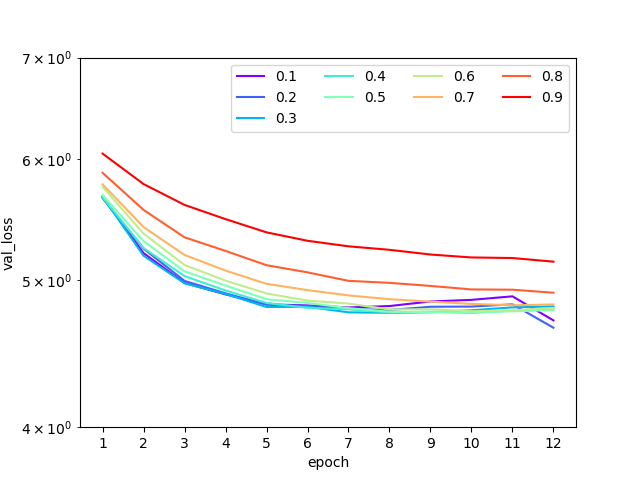Fig 12. awd-lstm-lm original implementation of AWD-LSTM language modelling on the Wikitext-2 dataset, varying a single dropout parameter between 0.1 and 0.9 keeping all other parameters at zero. a) Input Dropout (di), b) Hidden Dropout (dh), and c) Dropout (output dropout) (d).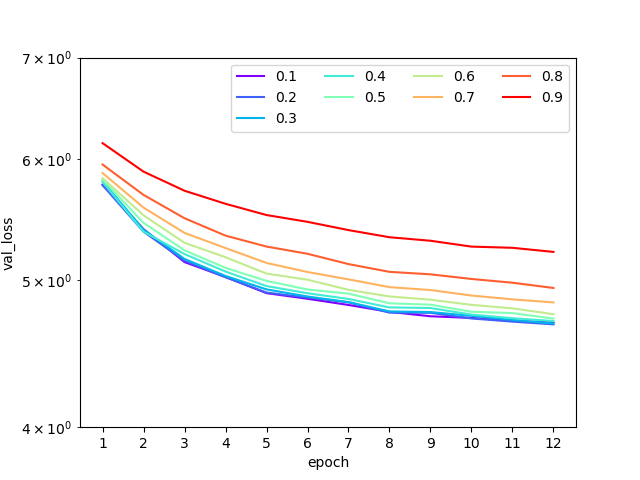Fig 13. awd-lstm-lm original implementation of AWD-LSTM language modelling on the Wikitext-2 dataset, varying a single dropout parameter between 0.1 and 0.9 keeping all other parameters at default values. a) Input Dropout (di), b) Hidden Dropout (dh), and c) Dropout (output dropout) (d).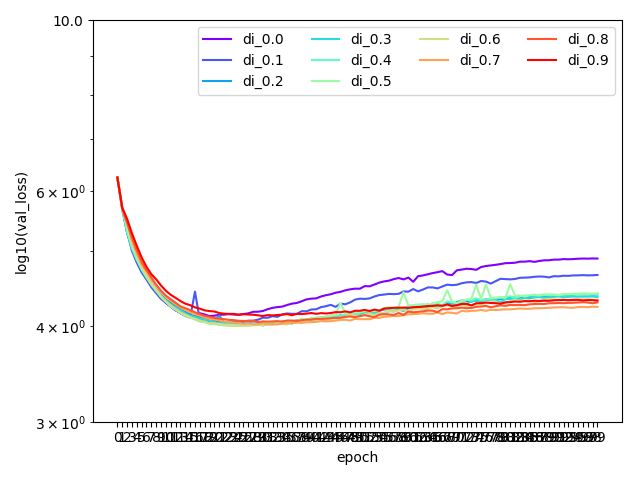Fig 14. a) AWD-LSTM (original) varying Input Dropout (di) parameter on PTB dataset. Other dropouts kept at default values. b) Fastai implementation of AWD-LSTM varying Input Dropout (di) parameter on PTB dataset. Other dropouts kept at default values.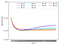Fig 15. a) AWD-LSTM (original) varying Hidden Dropout (dh) parameter on PTB dataset. Other dropouts kept at default values. b) Fastai implementation of AWD-LSTM varying Hidden Dropout (dh) parameter on PTB dataset. Other dropouts kept at default values.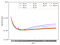Fig 16. a) AWD-LSTM (original) varying Output Dropout (d) parameter on PTB dataset. Other dropouts kept at default values. b) Fastai implementation of AWD-LSTM varying Output Dropout (d) parameter on PTB dataset. Other dropouts kept at default values.

Weight Drop (wdrop abbrv. wd) amount of weight dropout to apply to the RNN hidden to hidden matrix.

In the awd-lstm-lm codebase, each RNN is wrapped in a WeightDrop class during the initialization of the RNNModel.

`self.rnns = [WeightDrop(rnn, ['weight_hh_l0'], dropout=wdrop) for  rnn in self.rnns]`

Note how the hidden to hidden weights (weight_hh_l0) are only weights this is applied to. In the forward method in the WeightDrop class we call a _setweights() method which applies dropout to a ‘raw’ copy of the weights.

The Weight Drop parameter (wdrop) is a DropConnect (Wan et al. 2013) (as briefly summarized in part 1) type of dropout where rather than dropping activations, randomly selected weights themselves are dropped from the network during training.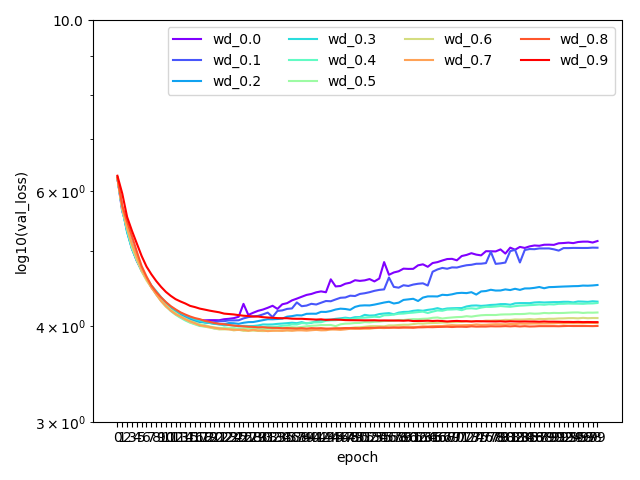Fig 17. a) AWD-LSTM (original) varying the Weight Drop (wd) parameter on PTB dataset. Other dropouts kept at default values. b) Fastai implementation of AWD-LSTM varying the Weight Drop (wd) parameter on PTB dataset. Other dropouts kept at default values.

With high weight drop for the original implementation of AWD-LSTM, loss values are off the charts in the figure above.

In the figures below I show the results running up to 12 epochs — a ‘zoomed in’ version of the plots above showing generally the fastest changing part of the loss vs epoch plot.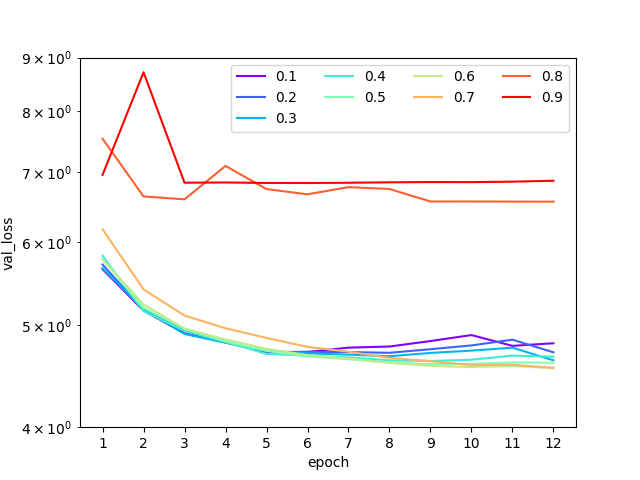Fig 18. awd-lstm-lm original implementation of AWD-LSTM language modelling varying the Weight Drop (wd) parameter on a) Wikitext-2 dataset and b) PTB dataset. All other dropout parameters are set to zero.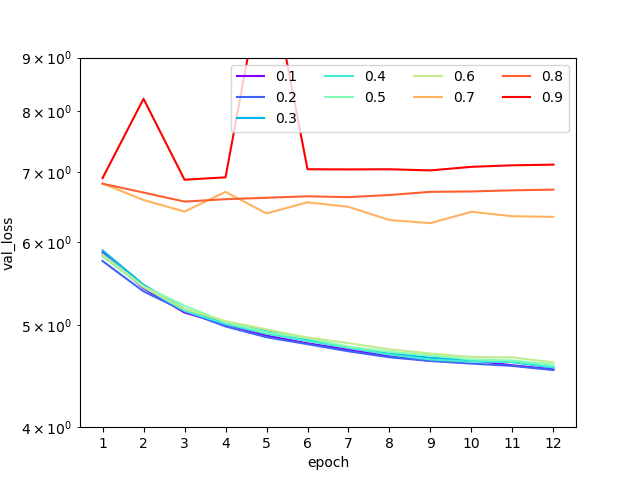Fig 19. awd-lstm-lm original implementation of AWD-LSTM language modelling varying the Weight Drop (wd) parameter on a) Wikitext-2 dataset and b) PTB dataset. All other dropout parameters are set to default values.

The most striking feature of the loss result as the weight drop parameter is varied are the two curve types, with a sudden shift in network performance at around 70% dropout. This response is not seen when running the same tasks on the fastai implementation of the AWD-LSTM code. A more detailed analysis on the reasons for this difference may be the subject of a follow-up post.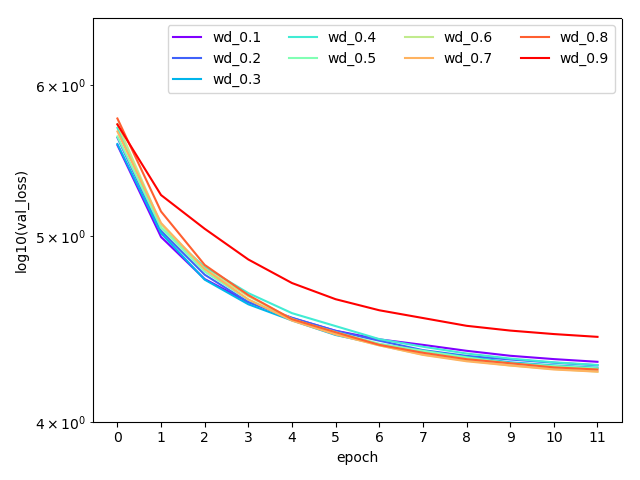Fig. 20. Fastai implementation of AWD-LSTM and variation of weight drop with other dropout parameters set to zero. a) Wikitext-2 language model (note y scale difference here to other plots). b) PTB language model.

Other parameters set to zero

Re-running the experiments for 100 epochs with other dropouts set to zero shows some interesting differences to when using defaults for other dropouts.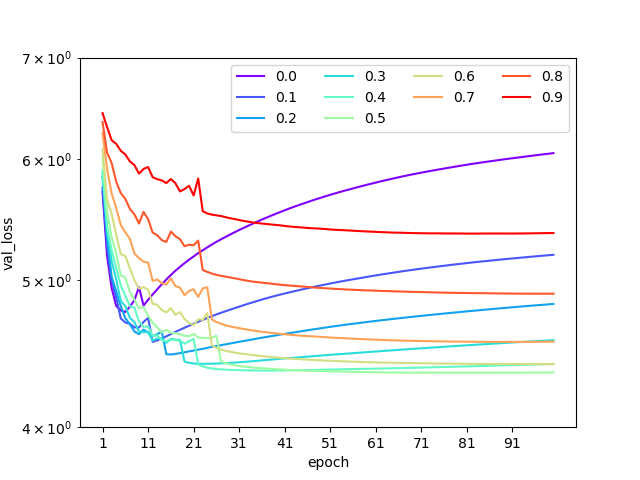Fig 21. awd-lstm-lm original implementation of AWD-LSTM on PTB dataset. a) Varying hidden dropout (dh), b) embedded dropout (de). Other dropout parameters are kept at zero.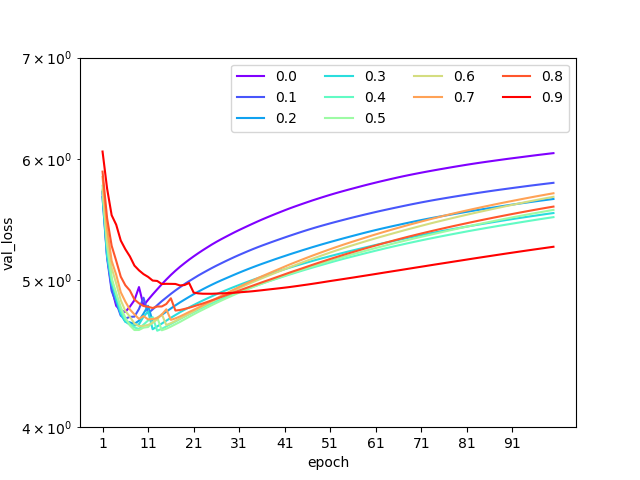Fig 21. cont. AWD-LSTM on PTB dataset. c) varying weight drop (wd), d) output dropout (d). Other dropout parameters are kept at zero.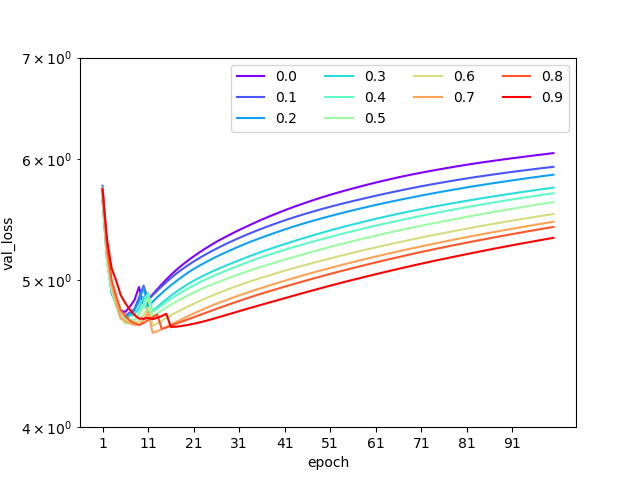Fig 21. cont. AWD-LSTM on PTB dataset. e) varying input drop (di). Other dropout parameters are kept at zero.

It is apparent that when just using a single dropout type (so far only output dropout (d), embedding dropout (de), hidden dropout (dh) and weight drop (wd) have been tested) results in deteriorating loss values after 10–20 epochs in most cases. If using AWD-LSTM, running 12 epochs and just applying dropout to embeddings will all other dropouts set to zero one might conclude that embedding dropout (de) should be set relatively low as per Fig. 6 above. Whereas best performance in this particular case would be with a embedding dropout of 0.5, with validation loss being quite sensitive to changes in embedding dropout.

In the tests below we see that like with the AWD-LSTM codebase, the fastai implementation of AWD-LSTM where a single dropout parameter is used with others set to zero most cases lead to increasing loss after 20 or so epochs.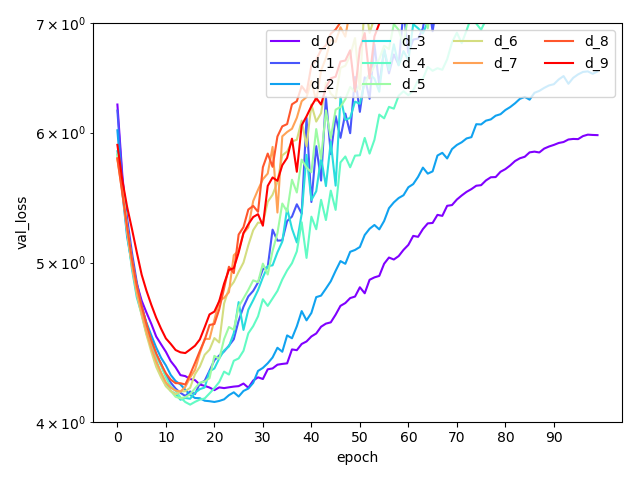Fig 22. Fastai implentation of AWD-LSTM on PTB dataset a) varying embedding dropout (de), b) varying weight drop (wd), c) varying outpout dropout (d). Other dropout parameters are kept at zero. Tests on other parameters with and without default values is in progress.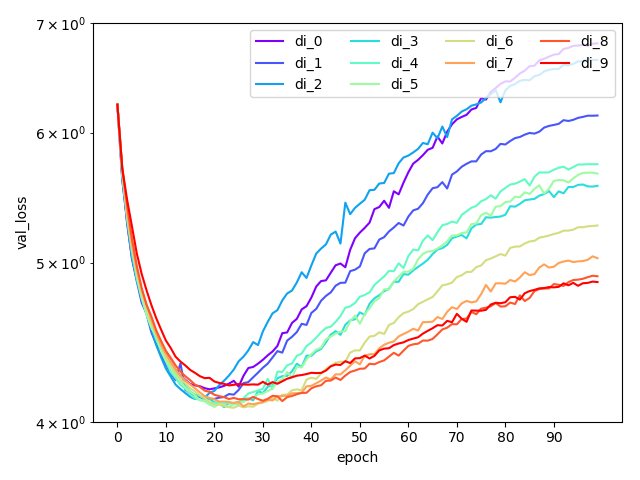Fig 22. cont. a) varying hidden dropout (dh), b) varying input drop (di). Other dropout parameters are kept at zero.

Preliminary findings of this work are that for language modelling with AWD-LSTM the Embedding Dropout the dropout with most range in resultant loss, with the input dropout having the least impact. Large Weight Drop values should not be used with the AWD-LSTM code base.

Sequence to Sequence Attention model for translation tasks

In this section I change from language modelling to translation task dropout analysis.

Here the task to to translate from one language to another. Here we use FastText pre-trained word vectors for both languages and the Shared Task: Machine Translation French-English training dataset (http://www.statmt.org/wmt15/translation-task.html).

Example question pairs in english and french are:

‘What is light ?’, ‘Qu’est-ce que la lumière?’

‘Who are we?’, ‘Où sommes-nous?’

In these tests the following dropout parameters were applied:

RNN Encoder Dropout (red): single dropout parameter applied to the RNN_Encoder. The dropout is applied as a default parameter for CudnnRNN()

`self.rnn_enc = nn.GRU(em_sz_enc, nh, num_layers=nl, dropout=rnn_enc_drop)`

RNN Decoder Dropout (rdd): single dropout parameter applied to the RNN_Decoder.

`self.rnn_dec = nn.GRU(em_sz_dec, em_sz_dec, num_layers=nl, dropout=rnn_dec_drop)`

Embedding Encoder Dropout (eed): single dropout parameter applied to input embeddings in the forward method of a sequence to sequence RNN (Seq2SeqAttnRNN in fastai code)

`self.emb_enc_drop = nn.Dropout(emb_enc_drop)...def forward(...)    ...    emb = self.emb_enc_drop(self.emb_enc(inp))`

Output Dropout (od): single dropout parameter applied to output in the forward method in a loop iterating over target language total number of sequences.

`self.out_drop = nn.Dropout(out_drop)...def forward(...)    ...    for i in range(self.out_sl):        ...        outp = self.out(self.out_drop(outp))`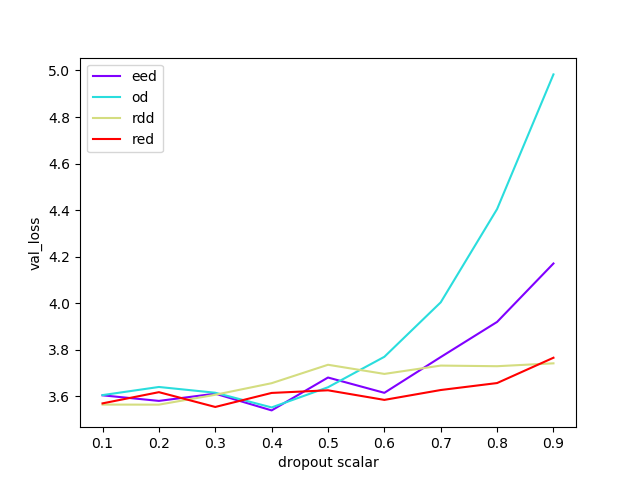Fig 23. Translation model using Sequence to Sequence RNN, impact of dropout parameters, spread of values for each parameter after 12 epochs.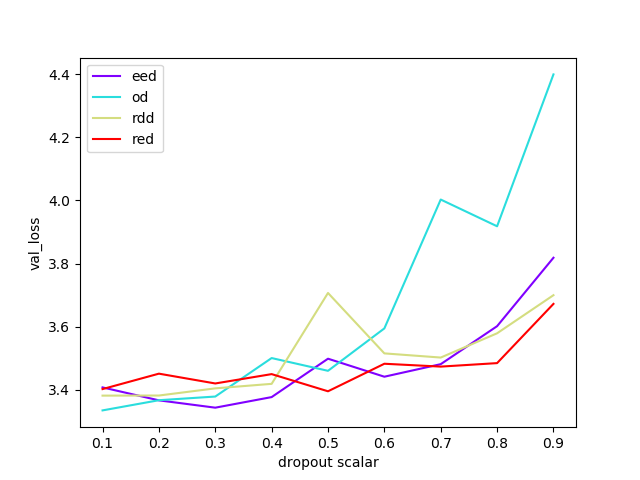Fig 24. Translation model using Sequence to Sequence Attention RNN, impact of dropout parameters, spread of values for each parameter after 12 epochs.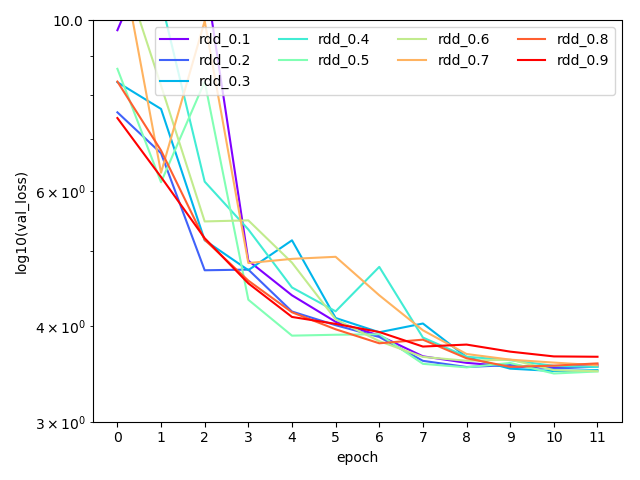Fig 25. Translation model using Sequence to Sequence Attention RNN. Individual dropout parameters a) red, b) rdd effects on loss with other dropout parameters set to zero.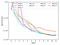Fig 26. Translation model using Sequence to Sequence Attention RNN. Individual dropout parameters a) od, b) eed effect on loss with other dropout parameters set to zero.

It is interesting to view the rate of change of the validation loss with epoch run as per below, showing we need to do a minimum of 7 or 8 epochs for change in validation loss to settle.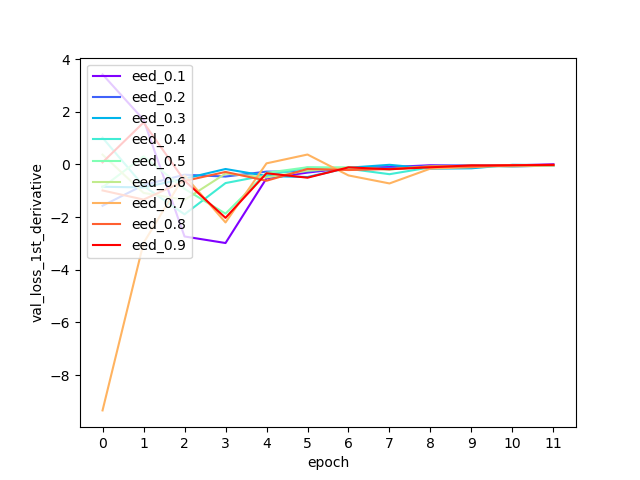Fig 27. Derivative of loss vs epoch for different dropout types.

The aim of this work was to get a feel for how different dropout types and how the amount of dropout applied for each type affects validation loss with recurrent neural networks. By understanding the effects of each parameter I can now focus on optimizing those with the greatest effect.

Appendix

Default dropout values (where defaults were used) for AWD-LSTM (awd-lstm-lm implementation) language modelling:

dropout = 0.4
dropouth = 0.2
dropouti = 0.65
dropoute = 0.1
wdrop = 0.5

Default dropout values for fastai code (where defaults were used), language modelling:

drops_scalar = 0.7
dropouti = 0.25*drops_scalar
dropout = 0.1*drops_scalar
dropoute = 0.02*drops_scalar
dropouth = 0.15*drops_scalar
wdrop = 0.2*drops_scalar

Geophysicist and Deep Learning Practitioner